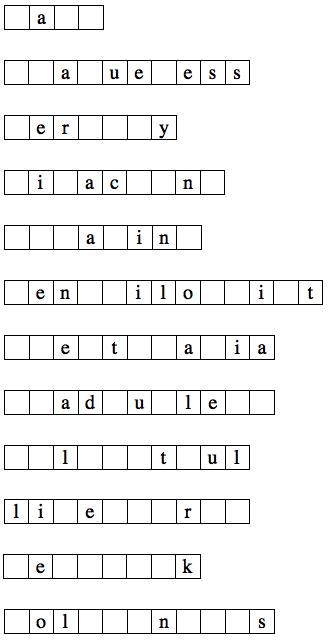Holiday Puzzles 2015

 1. CROSS ROADS For each set of 3 letters given, find a 6-letter word containing those letters in order, but not necessarily consecutively. Each row and column has a unique answer, but additional clues can be found using the intersecting words.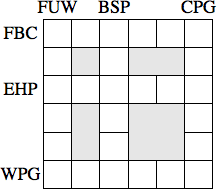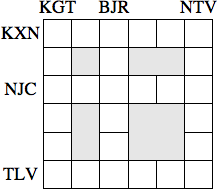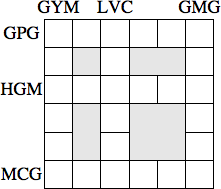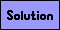2. HOLIDAY SPINNERS Divide the hexes into regions each containing exactly one dot, so that each dot is the center of rotational symmetry of its region.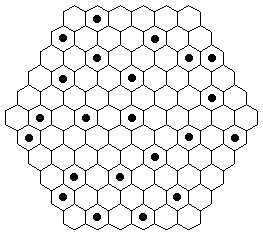3. THORNY CROWN Place the digits 1 through 9, each used exactly once, into the spaces provided. A number in a circle must be the first digit of the product of the neighboring spaces. A number in a square must be the last digit of the sum of the neighboring spaces.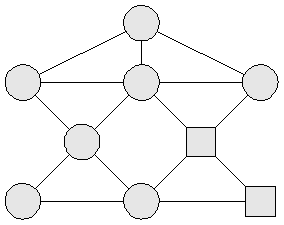4. DOMINOES In the figure to the right, all possible dominoes from 0-3 are shown so that each number is larger than the one below it, and different from the one to its left. Fill in the figures below with all possible dominoes from 0-4 according to the same restrictions.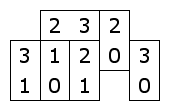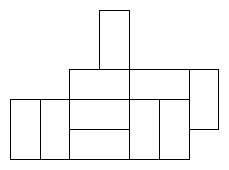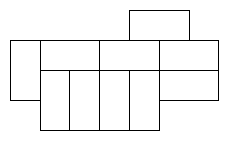5. CONSECUTIVE LETTERS Fill the blank squares in each word, one letter per square, with consecutive letters of the alphabet, but not necessarily in alphabetical order.